# Gauss's Law|Electric field due to continous charge distributions

## 1. Introduction

• Gauss's law was suggested by Karl Frederich Gauss(1777-1855) who was German scientist and mathematician.
• Gauss's law is basically the relation between the charge distribution producing the electrostatic field to the behavior of electrostatic field in space.
• Gauss's law is based on the fact that flux through any closed surface is a measure of total amount of charge inside that surface and any charge outside that surface would not contribute anything to the total flux.
• Before we look further to study Gauss's law in detail let's study electric field due to continuous charge distributions.

## 2. Electric field due to continous charge distributions

• So far as in the previous chapter we have discussed force and field due to discrete charges.
• We now assume that charges on a surface are located very close togather so that such a system of charges can be assumed to have continous distribution of charges.
• In a system of closely spaced charges, total charge could be continously distributed along some line, over a surface or throughout a volume.
• First divide the continous charge distribution into small elements containing Δq amount of charge as shown in fig 1

•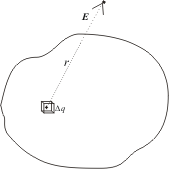• Electric field at point A due to element carrying charge Δq is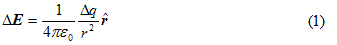where r is the distance of element under consideration from point A and rˆis the unit vector in the direction from charge element towards point A.
• Total electric field at point A due to all such charge elements in charge distribution is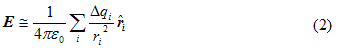where index i refers to the ith charge element in the entire charge distribution.
• Since the charge is distributed continuously over some region , the sum becomes integral. Hence total field at A within the limit Δq→0 is,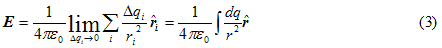and integration is done over the entire charge distribution.
• If a charge q is uniformly distributed along a line of length L, the linear charge density λ is defined by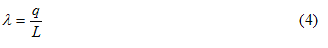and the unit of λ is Coulumb/meter(C/m).
• For charge distributed non-uniformly over a line, linear charge density is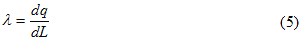where dQ is the amount of charge in a small length element dL.
• For a charge Q uniformly distributed over a surface of area A, the surface charge density σ is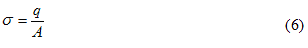and unit of surface charge density is C/m2. For non uniform charge distributed over a surface charge density is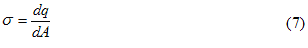where dA is a small area element of charge dQ.
• Similarly for uniform charge distributions volume charge density is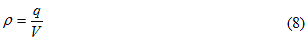and for non uniform distribution of charges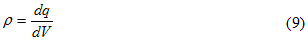and unit of volume charge distribution is C/m3.

## 3. Gauss's Law

• We already know about electric field lines and electric flux. Electric flux through a closed surface S is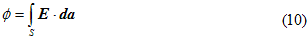which is the number of field lines passing through surface S.
• Statement of Gauss's Law
“ELectric flux through any surface enclosing charge is equal to q/ε0 , where q is the net charge enclosed by the surface”
mathematically,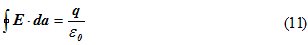where qenc is the net charge enclosed by the surface and E is the total electric field at each point on the surface under consideration.
• It is the net charge enclosed in the surface that matters in Gauss's law but the total flux of electric field Edepends also on the surface choosen not merely on the charge enclosed.
• So if you have information about distribution of electric charge inside the surface you can find electric flux through that surface using Gauss's Law.
• Again if you have information regarding electric fluxthrough any closed surface then total charge enclosed by that surface can also be easily calculated using Gauss's Law.
• Surface on which Gauss's Law is applied is known as Gaussian surface which need not be a real surface.
• Gaussian surface can be an imaginary geometrical surface which might be empty space or it could be partially or fully embedded in a solid body.
• Again consider equation 11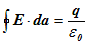In left hand side of above equation E·da is scalar product of two vectors namely electric field vector E and area vector da. Area vector da is defined as the vector of magnitude |da| whose direction is that of outward normal to area element da. So,da=nˆda where nˆ is unit vector along outward normal to da.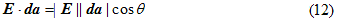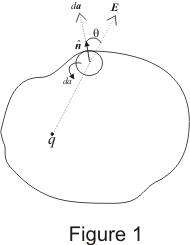From above discussion we can conclude that,
(1) If both E and surface area da at each points are perpendicular to each other and has same magnitude at all points of the surface then vector E has same direction as that of area vector as shown below in the figure.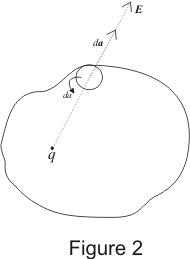since E is perpendicular to the surface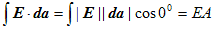(2) If E is parallel to the surface as shown below in the figure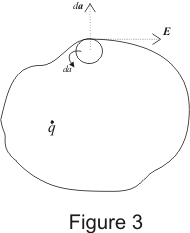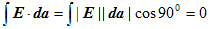and integral is also zero for zero value of electric field vector E at all points on the surface.